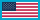Change Location# Battery Management Systems, Volume III: Physics-Based Methods

Pages: 360
ISBN: 9781630819040
Coming Soon: Available 08/31/2023
List Price: \$159.00

New Release Discount Price
Hardback \$119.00 Qty:

This book -- the third and final volume in a series describing battery-management systems – shows you how to use physics-based models of battery cells in a computationally efficient way for optimal battery-pack management and control to maximize battery-pack performance and extend life. It covers the foundations of electrochemical model-based battery management system while introducing and teaching the state of the art in physics-based methods for battery management.

Building upon the content in volumes I and II, the book helps you identify parameter values for physics-based models of a commercial lithium-ion battery cell without requiring cell teardown; shows you how to estimate the internal electrochemical state of all cells in a battery pack in a computationally efficient way during operation using these physics-based models; demonstrates the use the models plus state estimates in a battery management system to optimize fast-charge of battery packs to minimize charge time while also maximizing battery service life; and takes you step-by-step through the use models to optimize the instantaneous power that can be demanded from the battery pack while also maximizing battery service life.

The book also demonstrates how to overcome the primary roadblocks to implementing physics-based method for battery management: the computational-complexity roadblock, the parameter-identification roadblock, and the control-optimization roadblock. It also uncovers the fundamental flaw in all present “state of art” methods and shows you why all BMS based on equivalent-circuit models must be designed with over-conservative assumptions. This is a strong resource for battery engineers, chemists, researchers, and educators who are interested in advanced battery management systems and strategies based on the best available understanding of how battery cells operate.

1. Redundant Parameter Elimination

a. Introduction
b. Review of BMS definitions and tasks
c. Modeling approach #1: Empirical
d. Modeling approach #2: Physics-based
e. Reducing number of model parameters: method
f. Reducing number of model parameters: solid
g. Reducing number of model parameters: electrolyte, kinetics
h. Summary of reformulated PDEs
i. Recovering original electrochemical variables

2. Modeling Electrochemical Impedance

a. More detail required at the solid–electrolyte interface
b. Ideal interfacial impedance model
e. Seeking full-cell impedance model
f. Full cell impedance response
g. Nyquist (Cole–Cole) plots
h. MATLAB toolbox

3. Model Parameter Identification

a. Introduction
c. OCP testing
d. Initial data processing
e. Missing-data and inaccessible-lithium problems
f. Practical computation of differential capacity
g. Multi-species-multi-reaction (MSMR) model
h. Converting dis/charge data to OCP
i. Testing the methods using simulation data
j. Application to physical half cells
k. Correlating with cell-level OCV
l. Pulse-resistance testing
m. Frequency-response testing, temperature/SOC dependence
n. Distribution of relaxation times
o. DRT applied to cell impedance data
q. Lab-test procedures; data calibration; terminal resistance
r. Initialization of optimizations
s. Lumped-parameter constraints
t. MATLAB optimization scheme
u. Cost functions; results
v. MATLAB toolbox

4. Efficient Time-Domain Simulation

a. Introduction and context
b. Convert continuous-time to discrete-time frequency response
c. Illustrating frequency-response conversion method
d. Hybrid realization algorithm (HRA)
e. Final form of A , B , C , and D
f. Sample results
g. Simulating a (single) cell in the time domain, near a setpoint
h. Simulation results near a ROM setpoint
i. Simulating over a wide operating range (output blending)
j. Simulation results over wide operating range
k. Simulating constant voltage and constant power
l. Simulating battery packs
m. MATLAB toolbox

a. Introduction
b. Limitations on lithium-ion battery performance
c. Solid-electrolyte interphase (SEI) film growth
d. Identifying SEI-model parameter values
e. Lithium plating
f. MATLAB toolbox

6. Electrochemical Internal Variables Estimation

a. Introduction
b. Review of sequential probabilistic inference
c. The eight-step process
d. Approximating statistics with sigma points
e. SPKF with the output-blended model
f. Sample simulation results
g. MATLAB toolbox

7. Optimal Fast Charge

a. Fast-charge control problem
b. Limitations on fast-charging lithium-ion batteries
d. Model predictive control (MPC) – the basics
e. Applying MPC to fast-charge of lithium ion cells
f. MPC implementation
g. Simulation results
h. MATLAB toolbox

8. Power Limits Estimation

a. Survey on methods of power-limit calculation
b. MPC – a new paradigm for predictive power estimation
c. Power limit estimation: An MPC-inspired method
d. Power limit estimation – comparison of ECM & PBM
e. Summary and Next Steps
f. MATLAB toolbox

• Gregory L. Plett is a professor of electrical and computer engineering at the University of Colorado Colorado Springs. He received his B.Eng. in computer systems engineering from Carleton University in Ottawa, Ontario and his M.S. and Ph.D. in electrical engineering from Stanford University in Stanford, CA.
• M. Scottus Trimboli